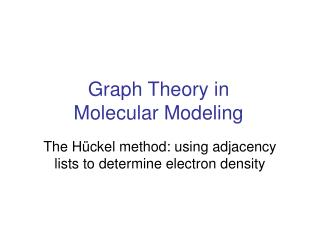DownloadDownload PresentationGraph Theory in Molecular Modeling

# Graph Theory in Molecular Modeling

Télécharger la présentation## Graph Theory in Molecular Modeling

- - - - - - - - - - - - - - - - - - - - - - - - - - - E N D - - - - - - - - - - - - - - - - - - - - - - - - - - -
##### Presentation Transcript

1. Graph Theory in Molecular Modeling The Hückel method: using adjacency lists to determine electron density

2. Graph Theory in Chemistry • Fullerenes are complex polyhedra • The number of pentagon-pentagon adjacencies help separate stable and unstable isomers. • Other graph invariants often match chemical properties: • Kekule counts help to qualitatively determine stability • Independence numbers serve as an indication of limits to chemical reactivity. • Adjacency eigenvalue spectrums determine electronic structure.

3. Molecular Orbitals(a really basic chemistry review) • Atoms are surrounded by electron clouds, represented by continuous density functions. • The smooth surfaces are really misleading

4. Molecular orbitals ii • Orbitals also extend over bonds between atoms—a [covalent] bond is fundamentally just shared electrons.

5. Molecular orbitals iii • Electron density over atoms and bonds is the driving force of chemical reactivity: • Determines charge & partial charge. • Each has an energy level, ergo thermodynamic reactions proceed. • Best tool for raw prediction

6. Hückelmethod • Use a weighted graph representation of a heteromolecule (or approximate one) • Atoms become vertices • Bonds become edges • We already draw them like this! • Include a number for the total edge weight • The number of electrons that make up our bonds • Add a set of functions we want to minimize • These functions will model electron repulsion

7. The hard part • A lot of equations, which are agonizing and I’m glossing over. • Basically, we have orbitals φμ and undetermined coefficients Cµi, for a given atom, orbital energies ε for a given orbital, and we get a ψi for each atom. • We want find values of Cµi to minimize E. This is the final equation.

8. Ugh, it gets worse • We can turn our previous constraints into a set of linear equations.* • New complexity requires approximations and simplification of the problem. * This requires solving the Schrödinger equation for a specific Hamiltonian, so I’m not going to cover it. If you’re curious, I can direct you to instructional sources.

9. I Hückel Hückel made it easier (an approximation): Now, we don’t need to even understand the form of the Hamiltonian (h), much less how it works.

10. Example & Demonstration • Ethane, an example from Australian Computational Chemistry via the Internet Project (ACCVIP). • There are a lot of programs, both commercial and free, that do all of this. A free visual one is the Simple Hückel Molecular Orbital (SHMO) calculator

11. Extensions • We’ve only accounted for heteromolecules. • Hückel assumes equal length bonds. • Other methods augment or replace Hückel: • CNDO/2 • Strict quantum chemical adherence • Fenske-Hall method